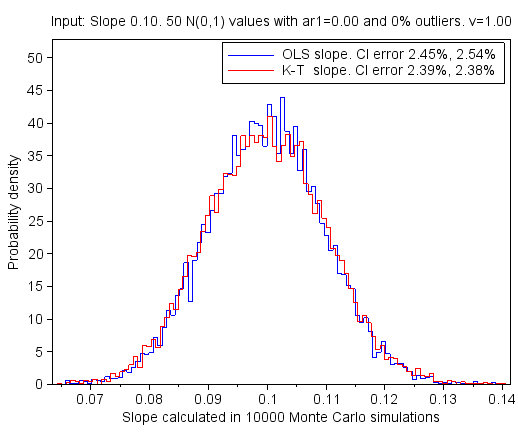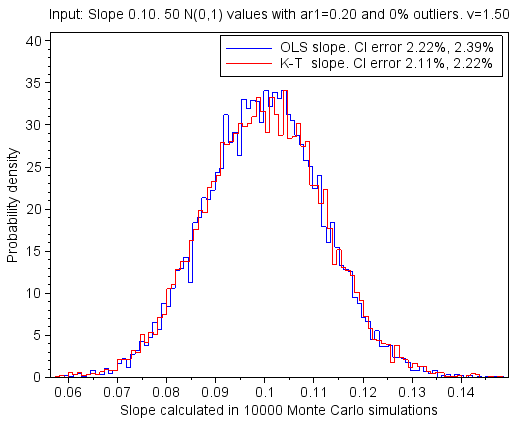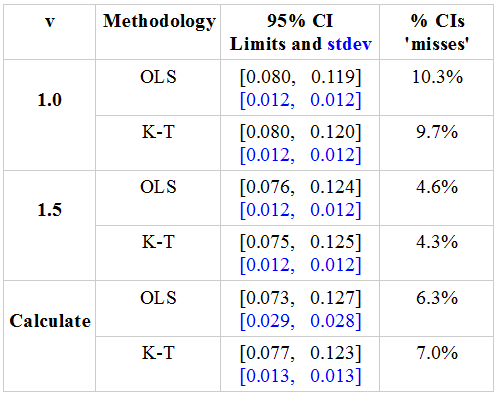## torsdag 9. mars 2017

### Compare Kendall-Theil and OLS trends

This is the fifth post in a series of six that deals with mathematics for calculation of correlation and trend in data with outliers. The posts are numbered 1 to 6. They should be read consecutively.

Post 1  Introduction to Statistical analysis of data with outliers
Post 2  Correlation when outliers in the data.
Post 3  Trend when outliers in the data.
Post 4  Correlation and trend when an outlier is added. Example.
Post 5  Compare Kendall-Theil and OLS trends.        Simulations.
Post 6  Detect serial correlation when outliers.               Simulations.

The posts are gathered in this pdf document.

### Start of post 5:  Compare Kendall-Theil and OLS trends

This blog post deals with Monte Carlo simulations that calculate trends. The calculations use both the Kendall-Theil (K-T) methodology and the Ordinary Least Squares (OLS) methodology. The post compares the results. Both methodologies work well when the noise in the dependent variable is white. They are about equal when there is serial correlation in the dependent variable. K-T is much more robust against outliers than OLS is. The results are presented in probability density plots.

All tests are done with a monotonic increasing x vector and a y vector with the data values. x may be the time and y may be some climate data. Both x and y have 50 items, which is a usual length in climate analysis. Each test is done in 10000 Monte Carlo simulations, with a new y vector in each simulation. The results of each test are shown as a probability density plot.

The independent variable x is the same in each simulation. The underlying trend in the dependent variable y is increasing with a slope of 0.1. Before each simulation a new noise vector is added to this underlying trend. The noise vectors in the same test have the same characteristics.

The noise vector is first filled with random numbers drawn from the standard normal distribution N(0,1). This is white noise. If the noise vector shall contain serial correlation, a first order Markov process with α equal to 0.20 is added to the vector. Thereafter, if the noise vector shall contain outliers, 4% of the noise values, randomly selected, are multiplied by 20. These definitions of serial correlation and/or outliers added to the noise are used throughout the blog post.

Random numbers are also called white noise. Serial correlation is also called coloured noise. A first order Markov process is also called an AR(1) process. The last section in this blog post, 5.5 Random noise, coloured noise and outliers, explains in more details how white noise, coloured noise and outliers are generated in the Monte Carlo simulations.

### 5.1 Only white noise

When the y vector contains white noise the K-T and the OLS methodologies both perform well, as shown in Figure 5.1.Figure 5.1: Probability density plot of the slopes calculated with Kendall-Theil (K-T) and Ordinary Least Squares (OLS).The noise in the dependent variable is white.

The mean value of the 10000 slopes calculated with both K-T and OLS is 0.100, and the standard deviation is 0.010. The probability density plots are almost identical, and they are both close to that of the normal distribution.

Each simulation calculates the slope and its 95% Confidence Interval (CI). In theory, it is 2.5% probability that the correct slope is less than the lower limit of the 95% CI, and it is 2.5% probability that the correct slope is larger then the upper limit of the 95% CI. We know the correct slope in our simulations. We can therefore calculate the percentage of the simulations in which the correct slope is less than the lower limit of the calculated 95% CI, and the percentage in which it is larger than the upper limit. These two percentages are displayed in the legend in Figure 5.1 as 'CI error'. The expected percentage is 2.5% for both. Large deviations from these expected values indicate that the methodology used in the calculations matches poorly with the nature of the noise.

The CI error percentages for both K-T and OLS in Figure 5.1 are close to the expected values. Both methodologies handle white noise well.

### 5.2 Outliers in the noise

Figure 5.2 shows that the Kendall-Theil methodology is more robust against outliers than the OLS methodology is. The mean values of the calculated slopes are close to the correct slope for both methodologies, but the spread of the calculated slopes is almost four times larger with the OLS methodology than it is with the K-T methodology.Figure 5.2: Probability density plot of the slopes calculated with Kendall-Theil (K-T) and Ordinary Least Squares (OLS).The noise in the dependent variable is white with outliers added to it.

The mean of the 10000 slopes calculated with K-T is 0.100, and the standard deviation is 0.011. The outliers caused only a minor increase in the standard deviation; from 0.010 to 0.011.

The mean value of the 10000 slopes calculated with OLS is 0.099 with standard deviation 0.041. The outliers cause a large increase in the standard deviation; from 0.010 to 0.041. This shows that OLS is not robust against outliers.

The probability density plot of the K-T slopes is close to that of the normal distribution with standard deviation 0.011, while the probability density plot of the OLS slopes is much thinner than that of the normal distribution with standard deviation 0.041.

The legend text in Figure 5.2 tells that the 95% confidence intervals of the OLS slopes are a little too narrow; 3.89% (1.87+2.02) of them do not cover the correct slope. The expected percentage is 5%. The 95% confidence interval of the K-T slopes is spot on, with 4.92% of them not covering the correct slope.

### 5.3 Serial correlation in the noise

Positive serial correlation in the y data means that parts of the noise in one measurement tend to be present also in the next measurement. Then parts of the noise in one measurement tend to be present also in the next measurement. This may be compensated for by calculating a multiplication factor v that is used to increase the uncertainty. For the OLS methodology the formula 2.4 in the post Hypothesis testing of temperature trends is used to calculate v, and for the K-T methodology the formula (3.13) in post 3 in this series is used.

I generate the serial correlation by adding a first order Markov process with α equal to 0.20 to the white noise in the y data. Then the expected lag 1 serial correlation coefficient is 0.2, and the expected lag 2 serial correlation coefficient is 0.04. With these coefficients the expected OLS v is 1.50 and the expected K-T v is 1.45.

The functions that calculate the OLS and the K-T trends can either calculate the compensation factor v individually for each set of 50 y elements, or they can use a preset value based on earlier experience and analysis. I recommend the latter approach, but for test purposes I first let the functions calculate the v value individually for each set of 50 y elements, and thereafter I calculate the mean value of them. The simulations with the OLS methodology gave a mean value of 1.75 with standard deviation 1.52. The corresponding numbers for the K-T methodology are a mean value of 1.29 with standard deviation 0.32. In these calculations, v values less than 1 are replaced with 1. This increases the mean value of v. We know from experience that we calculate too small serial correlation coefficients when we only have 50 y values in each data set. This decreases the mean value of v. Based on this I decide to use a preset v value of 1.5 for both OLS and K-T.Figure 5.3: Probability density plot of the slopes calculated with Kendall-Theil (K-T) and Ordinary Least Squares (OLS). The noise in the dependent variable is white plus a first order Markov process.

Figure 5.3 shows the probability density plots of the OLS and the K-T slopes. They are both calculated with the compensation factor v preset to 1.5. The v factor has large impact on the uncertainty of the calculated slopes, but it has no impact on the slopes themselves and therefore no impact on the probability density plots in Figure 5.3.

The mean of the calculated slopes is equal to the correct slope 0.100, and the standard deviation of the slopes is equal to 0.012. This is true for both OLS and K-T.

I repeated the Monte Carlo simulations with v equal to 1.0 (no compensation) and with calculation of v individually for each set of 50 y-values. The 95% confidence intervals for the slopes for these three v factors are shown in Table 5.1. They are shown both when calculated with the OLS and with the K-T methodology. I.e. the table shows the results from six Monte Carlo runs each consisting of 10000 simulations. The column % CI 'misses' shows the percentage of the confidence intervals that do not cover the correct slope. In approximately half of the misses the lower limit of the confidence interval is larger than the correct slope, and in the other half the upper limit is less than it.Table 5.1: The confidence intervals when there is serial correlation in the noise. The table shows how well the OLS and the K-T methodologies work when they try to compensate for the serial correlation. The first column shows the v factor used in the compensation, and the second column shows the methodology. The third column shows the mean and the standard deviation of the lower and the upper limits of the 95% Confidence Intervals in the 10000 Monte Carlo simulations. The means are in the upper line, and the standard deviations are in the second line in blue writing. The fourth column shows the percentage of the 95% confidence intervals that does not cover the correct slope. The expected percentage is 5%.

Table 5.1 demonstrates that it is necessary to compensate for serial correlation. When we do not compensate for it (v equal to 1.0), the calculated confidence intervals are too narrow; approximately 10 % of them do not cover the correct slope.

We get the most realistic confidence intervals when we compensate with v preset to 1.5. Then approximately 5% of the confidence intervals do not cover the correct slope.

The last row in Table 5.1 (Calculate) shows the results when we calculate v individually for each set of 50 y values. Then the confidence intervals are a little too narrow; between 6 and 7 % of them do not cover the correct slope. The v factor varies heavily depending on the y data set, despite that they have the same serial correlation when they were created. This approach is therefore dubious.

Table 5.1 shows that there is no great difference between the confidence intervals calculated by the OLS and the K-T methodologies. Both methodologies handle serial correlation in the noise well when they apply the preset factor of 1.5. The standard deviations of the limits in the 95% confidence intervals are in blue writing in the table. They are approximately 0.012 in all cases, except when the OLS methodology calculates v individually for each set of 50 y values. Then the standard deviation is more than twice as large. K-T is more robust than OLS in this case.

In the Monte Carlo simulations we know the answer with respect to serial correlation. With real data we do not know. Then the best approach is to evaluate the data, calculate v with many data sets, and thereafter conclude which v to be used as the preset value.

As an example, the monthly global temperature series have serial correlation. A warm month is usually followed by another warm month. For most of the monthly global temperature series we calculate a v factor around 12, meaning that the effective number of measurements approximately equals the number of years that the monthly series cover.

### 5.4 Both serial correlation and outliers in the noise

Now I add both serial correlation and outliers to the white noise. I do the Monte Carlo simulations with the same three approaches, as explained in the previous chapter, with respect to compensating for the serial correlation. That is without compensating for it (v=1), compensate for it with a preset compensation factor (v=1.5), and calculate the compensating factor individually for each set of 50 y values.

The probability density plots of the slopes calculated by the OLS and the K-T methodologies are shown in Figure 5.4. They are similar to those in Figure 5.2 when only outliers were added to the random noise.

The probability density plot of the K-T slopes is approximately normally distributed, while that of the OLS slopes is not. The mean of the slopes calculated with OLS and K-T are both equal to the correct value 0.100. With K-T the standard deviation of the slopes is 0.013, and with OLS it is 0.049, i.e. almost five times larger. This shows that K-T handles the outliers much better than OLS does, also when serial correlation is present in the noise.Figure 5.4: Probability density plot of the slopes calculated with Kendall-Theil (K-T) and Ordinary Least Squares (OLS). The noise in the dependent variable is white plus both a first order Markov process and outliers.

Table 5.2 shows how the choice of compensation for the serial correlation impacts the mean values and the standard deviations of the 95% confidence intervals in the simulations.Table 5.2: The confidence intervals when there is both serial correlation and outliers in the noise. The table contains similar information as Table 5.1, so see the explanation of that table.

The next paragraphs compare Table 5.1 and Table 5.2 to see how outliers, in addition to serial correlation, change the 95% confidence intervals.

When the K-T methodology is used, the outliers cause almost no change in the calculated 95% confidence intervals. But the intervals tend to be too narrow.

When the OLS methodology is used, the outliers cause the calculated 95% confidence intervals to be much wider than they are without the outliers. They tend to be too wide, most so when the v factor is preset to 1.5. Then only 1.7% of the intervals do not contain the correct slope.

The most realistic 95% confidence intervals in Table 5.2 are calculated with the K-T methodology when the v factor is preset to 1.5, and with the OLS methodology when the v factor is calculated individually for each set of 50 y values. In these two runs the K-T interval is [0.073, 0.128] and the OLS interval is [0.017, 0.182]. I.e. the OLS interval is three times wider than the K-T interval. Because both intervals are realistic, this shows that K-T is more robust against outliers than OLS is.

### 5.5 Random noise, coloured noise and outliers

This section explains how the noise vectors are generated.

The Monte Carlo simulations add noise to the data vectors before processing them. The standard normal distribution is the basis of the noise added, and we call it white noise. I use the Scilab rand() function to generate it.

Coloured noise may be added to the white noise. Then parts of the noise in one measurement tend to be present also in the next measurement. I implement the coloured noise as a first order Markov process.

Outliers may be added to the white noise. An outlier is much larger than the usual white noise. It is caused by either a measurement error or large variability in the data being measured.

White noise

We start with a noise vector x of length n. It contains white noise that follows a standard normal distribution N(0,1).

Coloured noise

When coloured noise shall be added to the noise, a first order Markov process is added to the noise vector x. The new x vector shall still have standard deviation 1, and we therefore reduce the original value of each x element before adding the coloured contribution to it. The coloured contribution is the factor α multiplied by the previous element, as shown in (5.1).

The factor α is between 0 and 1.

Outliers

When outliers shall be added to the noise, a percentage of the x values are increased by an outlier scale factor. The indexes of these x values are chosen at random. Even though only the values that were large before being multiplied by the factor become real outliers, I regard all the noise values in x that are multiplied by the factor as outliers.

Reference for the mathematics

Chapter 6.3 in the document Time series analysis published by the Wright State University shows how a first order Markov process is generated. The expected lag 1 serial correlation coefficient of the modified x vector in (5.1) is α, the lag 2 coefficient is α2, and so forth.

Previous and Next post in the series